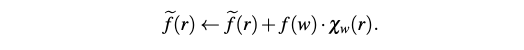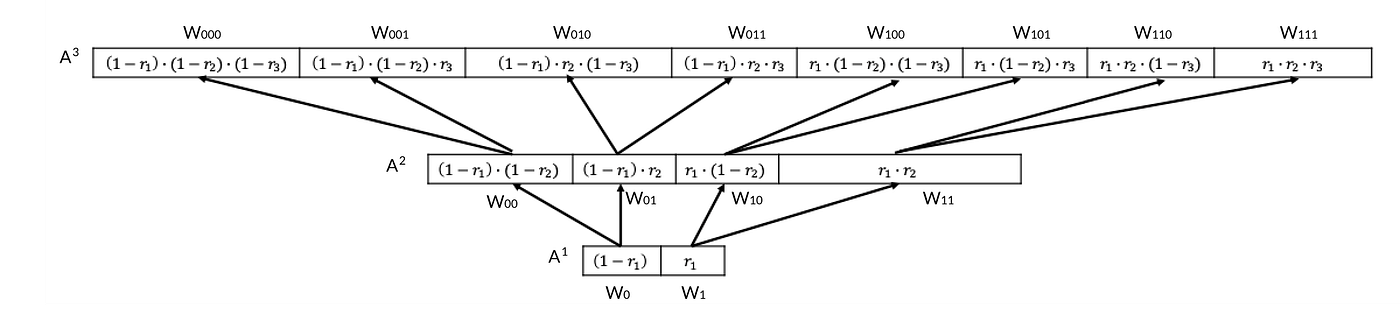# Rust Guide: Evaluating multilinear extensions via Lagrange interpolation

## This is an unofficial & unaudited Rust implementation of algorithms in Dr. Thaler’s Proofs, Arguments, & ZK book.

This week we learned why multilinear extensions are useful for interactive proofs.

In short, and in egregious laymen terms, multilinear extensions give us the ability to both (1) distance amplify a polynomial `f` , into `f~`, which can be evaluated by the Verifier and (2) still keep the new `f~` as a low degree polynomial.

(1) ensures that prover can’t convincingly lie about knowing `f` , by just providing random evaluations on some other bad `f’`

(2) ensures efficient runtimes for both Prover and Verifier operations

# Walkthrough

Let’s implement the Lagrange interpolation of multilinear polynomials 3 different ways.

To follow the notation in the following tutorial, please reference starting lemma 3.6 in Dr. Thaler’s book linked here.

The full implementation with tests & benchmarking can be found here:

Necessary caveat: I’m neither a mathematician nor a Rust expert. Suggestions for improvement are always welcome! 🥰

## Method 1: slow

In a rote approach, we stream over the evaluation table `f(w)` and sum up the resulting computation on each evaluation element.

Within the computation for each evaluation, we first retrieve the correct notation of `w` in `{0,1}^v`. For example, the following gives us `8->[1,0,0,0]`

Then we compute its χ by iterating over the vector `w` and `r` (verifier input), calculating each step of χ:`(xiwi +(1−xi)(1−wi))` at all vector positions`i`.

This implementation is `O(v* 2^v)`. When benchmarked using a function `f` mapping `{0, 1}^2` to the field F5, we get a median runtime of 1,402 ns per iteration.

## Method 2: stream (lemma 3.7)

Lemma 3.7 presents a more space efficient way of streaming over f(w), which can be achieved by making `slow_mle` recursive.Source: Proof of Lemma 3.7 in https://people.cs.georgetown.edu/jthaler/ProofsArgsAndZK.pdf

This is implemented as:

And to invoke this, we simply do:

This implementation is also `O(v* 2^v)` runtime but with a space improvement at `O(logn)`.

When benchmarked using a function f mapping `{0, 1}^2` to the field F5, we get a median runtime of `1,312 ns` per iteration.

## Method 3: dynamic (lemma 3.8)

Lemma 3.8 memoizes the computations χ and provides it as a look up table later used in the (figure 3.1) summation step.

The intuition is that, for example, the χ for `w=[0,0,0]` & `w=[0,0,1]`both involve calculating χ for `w=[0,0]`.

In fact, the χ for `w=[0,0,0]` is just `χ for w= times (1-r_i)`.A modified visual of figure 3.3 in https://people.cs.georgetown.edu/jthaler/ProofsArgsAndZK.pdf

So we can memoize and avoid recomputing intermediate χ values as we build the lookup table.

Here, we use recursion to achieve memoization.

Finally, we invoke the function to build the χ look up table and compute the inner dot product of `f~` (figure 3.1).

This implementation only has a linear runtime of `O(2^v)`, albeit with a space utilization of `O(n)`.

Benchmarked against methods 1 and 2, method 3 yields a huge runtime improvement (for v=2 on a field F5).